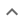•# Factoring multivariable polynomials

We think you wrote:

###This solution deals with factoring multivariable polynomials.

Solution found(3a-5b)*(a+3b)

## Step by Step Solution## Step  1  :

#### Equation at the end of step  1  :

  ((3 • (a2)) +  4ab) -  (3•5b2)
Step  2  :

#### Equation at the end of step  2  :

  (3a2 +  4ab) -  (3•5b2)


## Step  3  :

#### Trying to factor a multi variable polynomial :

Factoring    3a2 + 4ab - 15b2

Try to factor this multi-variable trinomial using trial and error

Found a factorization  :  (3a - 5b)•(a + 3b)

## Final result :

  (3a - 5b) • (a + 3b)